Today we'll be taking a look at resolving forces. This is a topic that you'll encounter in a mechanics math module, and it can be tricky to get your head around at first - but really we're just reusing some GCSE concepts. To get started, we'll revise some of the core concepts that we'll need to be familiar with, before moving onto some trickier problems.

Before moving on, try and make sure your mathematics knowledge includes a sound understanding of algebra.

## Gravity

It's pretty important to remember a few key facts about gravity. We normally say that gravity's acceleration is 9.8ms^-2. This is the case on earth, and often this figure is just substituted for the symbol "g".

We're also going to need to be familiar with forces. From physics, you may remember that F = ma. That is, a force (in Newtons) is equal to the mass of an object multiplied by its acceleration.

We've just alluded to how, really, gravity is just an acceleration. With that in mind, we can tweak our equation above slightly to make this new one:

W = mg

That is: weight is equal to the mass of an object, multiplied by the acceleration of gravity. You can probably use your mathematical prowess to see how really, this is algebraically the same equation, just using a specific acceleration (gravity). This also means that weight is a force.

## Trigonometry

There's one final thing you'll need to have a hold on before we get started, and that's our basic trigonometric functions: SOHCAHTOA. You can read up on them here.

We're going to need these because resolving forces will involve splitting each force up into an x (horizontal), and y (vertical) component, but more on that below.

## Resolving forces

Let's get started resolving some forces! Our question is going to be theoretical, as we don't want to complicate it too much, but quite often you'll find these questions involve some sort of scenario, and the key is to boil this down to a set of numbers that you can solve for.

Remember: always draw a diagram!

Let's say there are two forces acting on an object. One force acts horizontally, with a magnitude of 50N, while another force acts at an angle of 20 degrees, with a magnitude of 20N. What is the resultant ("overall") force acting on this object?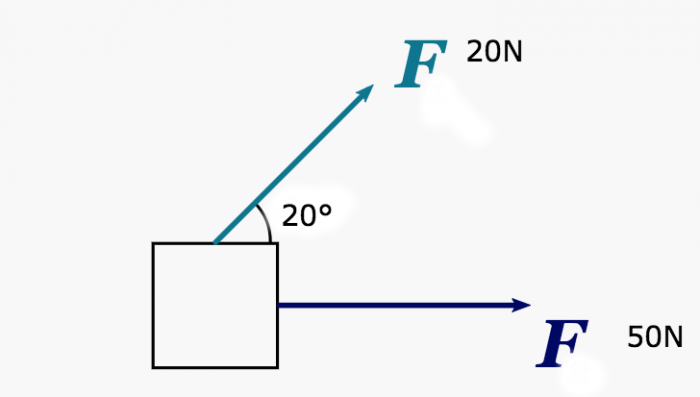Now, because these two forces are acting in different directions, we can't simply add them together and get resultant force. Remember, "resultant" is just a fancier word for saying overall, or total.

Our force of 50N is only acting in one direction (horizontally), so there's not too much to do here. We'll be focusing on the force of 20N that's acting at an angle of 20 degrees. Just like I mentioned before, we're going to need to split this one into its component forces (that is, the amount it acts horizontally (on the x axis), and the amount it acts vertically (on the y axis)). Don't worry, it's not too difficult!

If we imagine our 20N force acting at 20 degrees as a triangle, then we'd have a right angle triangle - with an acute angle of 20 degrees. The hypotenuse of the triangle would be 20N.

Using SOHCAHTOA and some simple algebra, we can calculate the forces that act on our adjacent and opposite triangle sides. We'll start by working out the horizontal force, which acts on the x axis, and is the adjacent side of our triangle.

Using SOHCAHTOA:

We know that our angle is 20, and that the hypotenuse is also 20. With some shifting around, we can re-arrange this equation to find the magnitude of our force in the horizontal direction: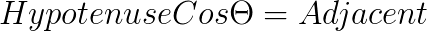And if we substitute our numbers in: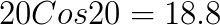Our force is acting at 18.8 Newtons in the horizontal direction.

Now, to find out the amount of force acting in the vertical direction, we can do a very similar calculation, just using sin instead of cos.

From SOHCAHTOA:

Again, using our algebra knowledge we can rearrange this equation, except this time we'll be finding the opposite side of the triangle. The opposite side is the side that is vertical, and represents our force's magnitude on the y axis.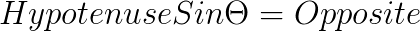Again, we already have our angle (which theta represents), and the hypotenuse, so we can just substitute those in to find the force acting vertically: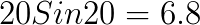Great! The force acting vertically from our original 20N force at an angle of 20 degrees is 6.8N!

## Piecing everything together

So, at this point, we know that there was a force of 50N acting horizontally on our object, and a force of 20N acting at a 20-degree angle. We've just split that 20N force into a 6.8N vertical force, and an 18.8N vertical force.

To find our resultant force, we'll just need to add these values together.

## Vertical forces

We'll start with vertical forces. This is going to be pretty easy:

6.8N + 0 = 6.8N

Yep, that's right: our 50N horizontal force doesn't act at all in the vertical direction, so the amount of force there is 0. That just leaves us with the vertical component of our 20N force.

### Horizontal forces

This one is going to be a little more complex, but still pretty intuitive:

50N + 18.8N = 68.8N

We take our 50N horizontal force and add it to the horizontal component of the 20N force. That leaves us with a total force of 68.8N on the X axis.

### A final triangle

So, we now have the 2 components of our resultant force, but not the overall force. That is, we know the resultant force horizontally, and the resultant force vertically, but we don't know what they are combined. To solve this, we can use one last triangle.

Place your horizontal force of 68.8N on the adjacent side of the triangle, and your vertical force of 6.8N on the opposite side of the triangle.

How can we calculate the combined force? Using our trusty triangular equation... Pythagoras' theorem! We have two sides of the triangle, and to calculate the hypotenuse is just a case of:

Let's substitute in our values and finish solving the overall resultant force: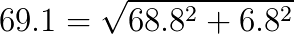And there we have it: a resultant force of 69.1N.

### Bonus point!

A final question you may be asked is solving the angle of the resultant force. Now that we have all three sides of the triangle, this is made easy with SOHCAHTOA.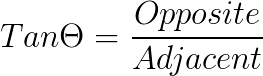To find the angle, we just need to use the inverse tan function.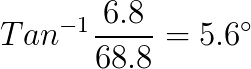And there we have it: our resultant force acts at an angle of 5.6 degrees.

## Summary

We hope you found this article informative. We'd recommend you keep studying using some past exam questions or worksheets that present this problem as we have above, and then some that use a scenario like calculating the forces acting on a picture frame. Remember: revision is the key to succeeding in maths exams! Once you understand the principles, each question is the same, because the curriculum only sets out certain subjects to study.

It's important to note that we haven't considered any friction in this example, but this may come up in an exam. If you feel like you need more help with resolving forces as part of your mechanics work, we'd recommend finding a maths tutor on Superprof to help you study!

Using maths tutors helps you grasp concepts you may have missed in the classroom, and allows subjects to be taught in a way that suits you.

You'll be a mathematician in no time!

For yet more tricky Maths problems explained, visit these guides on Calculus and Exponentials and Logs.

Need a Mathematics teacher?0 vote(s)Loading...